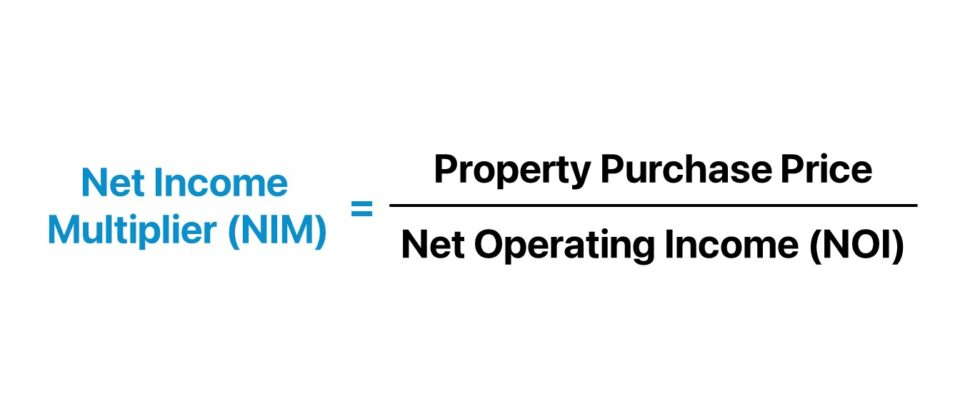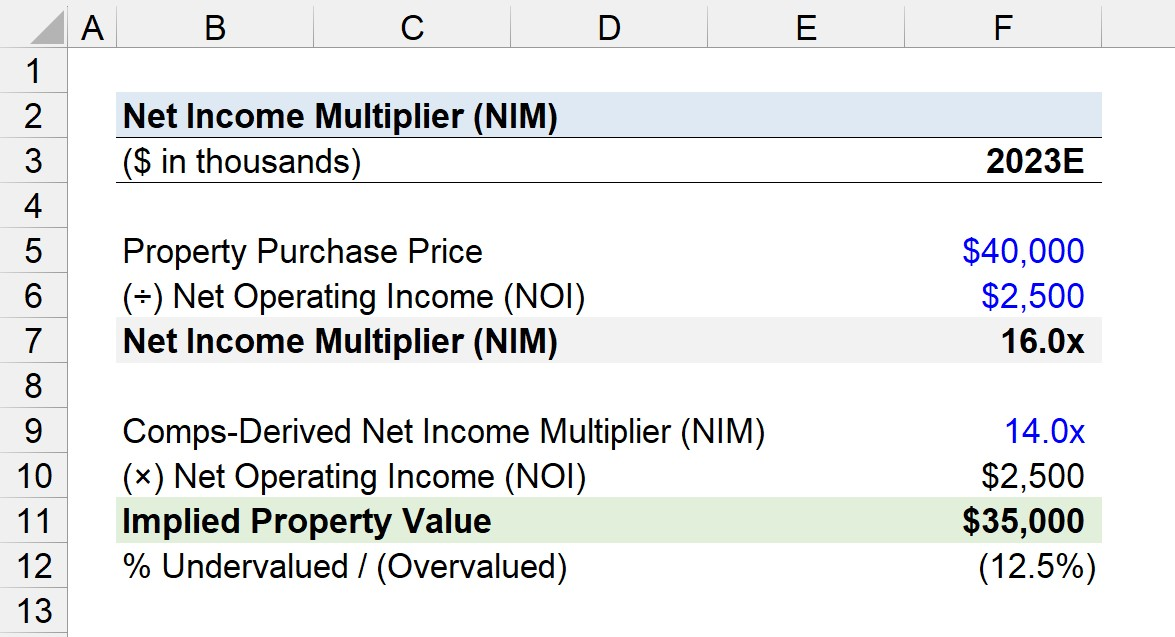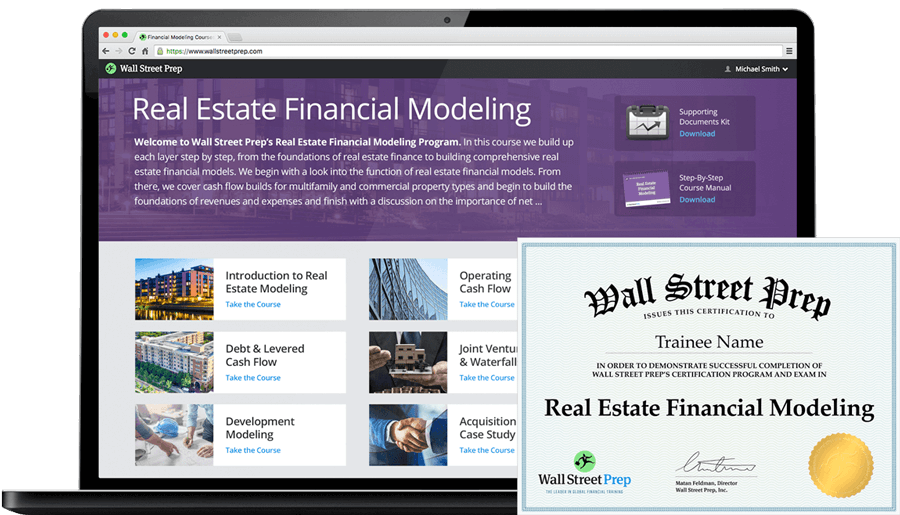Welcome to Wall Street Prep! Use code at checkout for 15% off.# Net Income Multiplier (NIM)

Guide to Understanding Net Income Multiplier (NIM)## How to Calculate the Net Income Multiplier?

In the real estate industry, the net income multiplier (NIM) is an investing metric calculated as the ratio between the purchase price of a given property and its annual net operating income (NOI).

• Property Purchase Price → The price at which an investment property can be acquired by a buyer in the market.
• Net Operating Income (NOI) → The rental and ancillary income generated by a property before deducting operating expenses and debt costs, such as mortgage payments and periodic interest obligations.

Similar to the gross rent multiplier (GRM), gross income multiplier (GIM), and the capitalization rate (or “cap rate”), the primary use-case of the net income multiplier (NIM) is for estimating the price of a property using a comps-derived multiplier.

For rental property investments, most of the income stream earned by the real estate investor is comprised of rent payments collected from tenants as part of a leasing arrangement.

Because the net income multiplier uses net operating income (NOI) in the denominator, the NIM method is a more practical approach to perform comps analysis on similar properties on a more “apples-to-apples” basis.

The process of calculating the net income multiplier (NIM) is a three-step process:

1. Identify Property Purchase Price → The first step is to identify the current sale price of the property, as of the most recent date, which is typically readily available information found online, i.e. the listing price.
2. Compute Net Operating Income (NOI) → The net operating income (NOI) is determined by taking the property’s effective gross income (EGI) and subtracting any direct operating expenses.
3. Calculate Net Income Multiplier (NIM) → In the final step, the net income multiplier is computed by dividing the property purchase price by the property’s net operating income (NOI).

## Net Income Multiplier Formula (NIM)

The formula to calculate the net income multiplier (NIM) consists of dividing the purchase price of a property by its net operating income (NOI).

Net Income Multiplier (NIM) = Property Purchase Price ÷ Net Operating Income (NOI)

Where:

• Property Purchase Price → The current stated selling price of the property on the market for sale (i.e. the listing price)
• Net Operating Income (NOI) → The income generated by a property across a one-year period after subtracting operating expenses from its effective gross income (EGI).

Like the gross rent multiplier (GRM), the valuation of a property can be determined using the net income multiplier (NIM).

Given the net income multiplier (NIM), the value of a real estate property can be estimated as the product of the multiplier and the property’s net operating income.

Estimated Property Price = Net Operating Income (NOI) × Net Income Multiplier (NIM)

## Net Income Multiplier vs. Gross Rent Multiplier: What is the Difference?

The distinction between the net income multiplier (NIM) and gross rent multiplier (GRM) is as follows.

• Net Income Multiplier (NIM) → The net income multiplier (NIM) measures a property’s valuation by comparing its purchase price to its net operating income (NOI). In relation to the gross rent multiplier, the net income multiplier is viewed as a more conservative metric since operating expenses are deducted.
• Gross Rent Multiplier (GRM) → The gross rent multiplier (GRM) is another method to measure the value of rental property investments. The GRM is calculated by dividing a property’s purchase price (or market value) by its gross annual rental income.

## Net Income Multiplier vs. Cap Rate: What is the Difference?

The net income multiplier (NIM) measures the number of times higher the purchase price of a property fares versus the property’s net operating income (NOI).

In contrast, the cap rate – or “capitalization rate” – is a fundamental return metric used among real estate practitioners to estimate the potential yield on property investments.

The cap rate describes the relationship between the net operating income (NOI) of a property and its purchase price (or market value) while providing useful insights into the property’s income potential.

Conceptually, the net income multiplier (NIM) is the reciprocal of the cap rate – or, more specifically, the “going-in” cap rate.

Cap Rate (%) = Net Operating Income (NOI) ÷ Purchase Price

Therefore, from the perspective of a real estate investor, a lower net income multiplier (NIM) – the reciprocal of the cap rate – is perceived to be more favorable to investors in terms of selecting a potential investment with an attractive risk-reward profile.

Why? The property’s annual net operating income (NOI) constitutes a higher percentage of the purchase price paid to acquire said property.

Note: The cap rate formula can use the market value of the property in the denominator rather than the purchase price, however, using the purchase price is more appropriate here for illustrative purposes.

## Net Income Multiplier Calculator (NIM)

We’ll now move to a modeling exercise, which you can access by filling out the form below.#### Get the Excel Template!Submitting...

## Net Income Multiplier Calculation Example (NIM)

Suppose a commercial property building is currently on sale for \$40 million.

In 2023, the building is anticipated to reach stabilization and generate \$2.5 million in net operating income (NOI).

• Property Purchase Price = \$40 million
• Net Operating Income (NOI) = \$2.5 million

The net income multiplier can be determined by dividing the purchase price of the property by its NOI, which comes out to a net income multiplier of 16.0x.

• Net Income Multiplier (NIM) = \$40 million ÷ \$2.5 million = 16.0x

In comparison, we’ll assume the comps-derived net income multiplier (NIM) of comparable properties is 14.0x, which is obtained via performing market research.

• Comps-Derived Net Income Multiplier (NIM) = 14.0x

Upon applying the 14.0x net income multiplier by the property’s NOI, we arrive at an implied property value of \$35 million, which suggests the current sale price is overvalued by approximately \$5 million (or by 12.5%).

• Implied Property Value = 14.0x × \$2.5 million = \$35 million
• % Undervalued / (Overvalued) = (\$35 million ÷ \$40 million) – 1 = (12.5%)20+ Hours of Online Video Training

### Master Real Estate Financial Modeling

This program breaks down everything you need to build and interpret real estate finance models. Used at the world's leading real estate private equity firms and academic institutions.

Inline Feedbacks